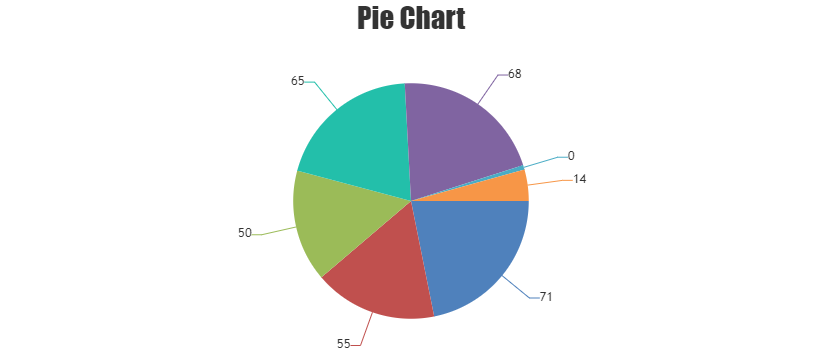Home Forums Chart Support Canvas Pie Chart

# Canvas Pie Chart

Viewing 2 posts - 1 through 2 (of 2 total)
• #35230

Is it possible to show slight/small bar for zero Y value in the pie chart.?

#35264

Sivaranjani

It’s not possible to show slice with a minimum space in pie-chart when the datapoint value is 0. However you can either show indexlabel to the section or add a defined value as minimum value to it as shown in below code-snippet.

``````function setMinValue() {
var data = chart.options.data;
for(var i = 0; i < data.length; i++) {
for(var j = 0; j < data[i].dataPoints.length; j++) {
var yValue = data[i].dataPoints[j].y;

if(yValue <= minValue && yValue >= -minValue) {
data[i].dataPoints[j].y = yValue < 0 ? yValue : minValue;
data[i].dataPoints[j].toolTipContent = yValue.toString();
data[i].dataPoints[j].indexLabel = yValue.toString();
}
}
}
}``````

Please take a look at this JSFiddle for complete code.Viewing 2 posts - 1 through 2 (of 2 total)

You must be logged in to reply to this topic.